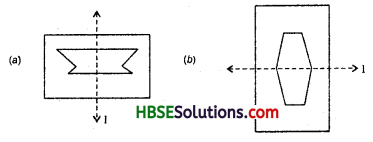# HBSE 6th Class Maths Solutions Chapter 13 Symmetry Ex 13.2

Haryana State Board HBSE 6th Class Maths Solutions Chapter 13 Symmetry Ex 13.2 Textbook Exercise Questions and Answers.

## 13.2

Question 1.
Find the lines of symmetry for each of the following shapes.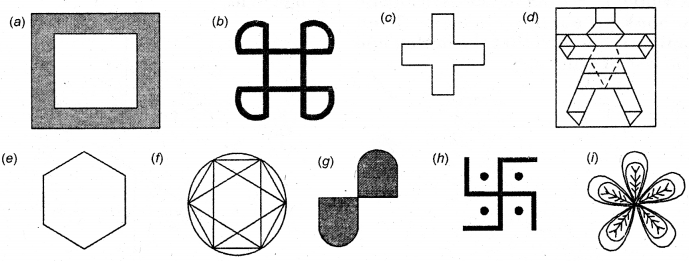Solution: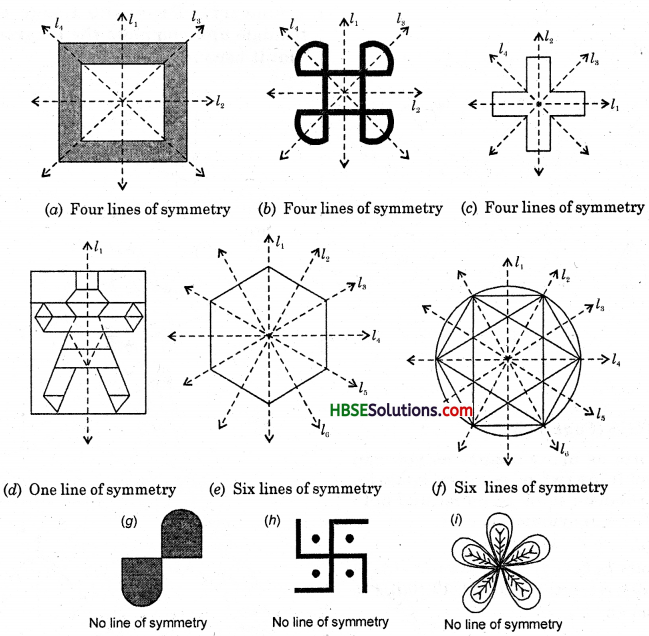Question 2.
Copy the triangle in each of the following figures, on squares paper. In each case draw the line(s) of symmetry if any and identify the type of triangle. (Some of you may like to trace the figures and try paper-folding first.)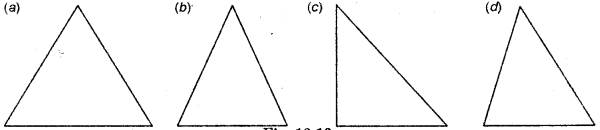Solution: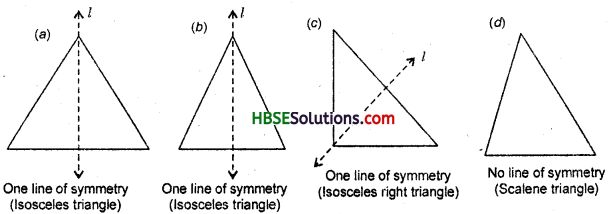Question 3.
Complete the following table :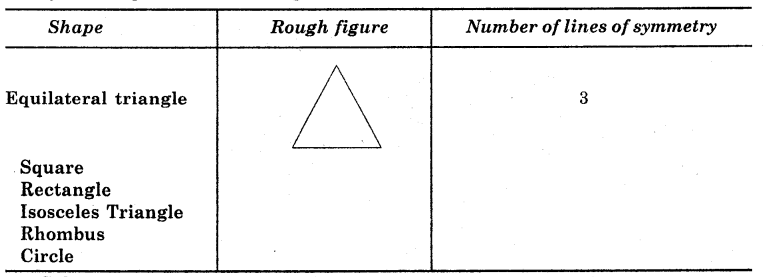Solution: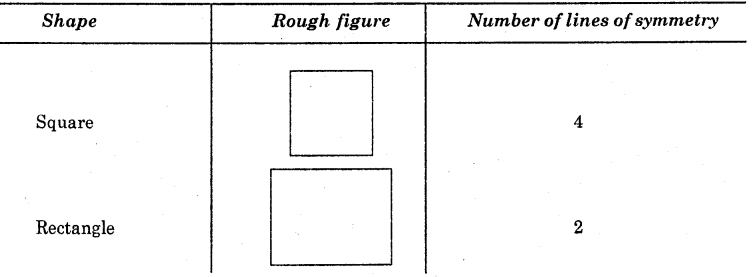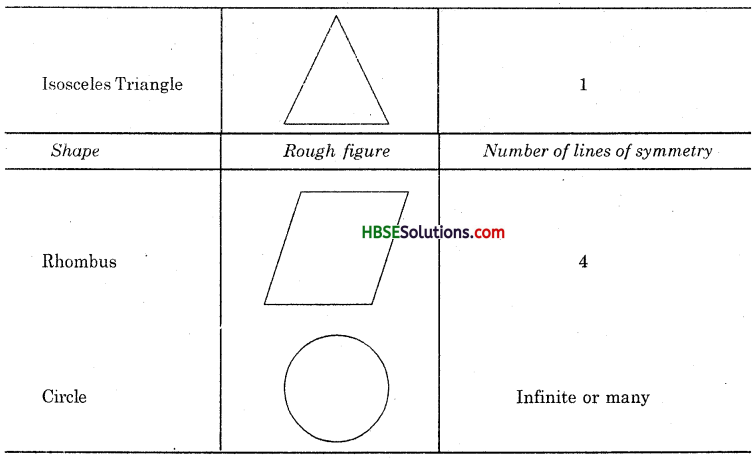Question 4.
Can you draw a triangle which has
(a) exactly one line of symmetry ?
(b) exactly two lines of symmetry ?
(c) exactly three lines of symmetry ?
(d) no lines of symmetry ?
Sketch a rough figure in each case.
Solution:
(a) An isosceles triangle has exactly one line of symmetry.
(b) We cannot draw a triangle which has exactly two lines of symmetry.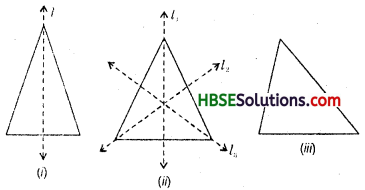(c) An equilateral triangle has exactly three lines of symmetry.
(d) A scalene triangle has no lines of symmetry.

Question 5.
On a squared paper-sketch the following :
[Hint: It will help if you first draw the lines of symmetry and then complete the figures.]
(a) A triangle with a horizontal line of symmetry but no vertical line of symmetry.
(b) A quadrilateral with both horizontal and vertical lines of symmetry.
(c) A quadrilateral with a horizontal line of symmetry but no vertical line of symmetry.
(d) A hexagon with exactly two lines of symmetry.
(e) A hexagon with six lines of symmetry.
Solution:
(a) An isosceles triangle has one line of symmetry. [Fig. (i)]
(b) A rectangle has two lines of symmetry. [Fig. (ii)]
(c) A trapezium has one line of symmetry. [Fig. (iii)]
(d) In Fig.(iv), a hexagon has exactly two lines of symmetry.
(e) A regular hexagon has six lines of symmetry. [Fig. (v)]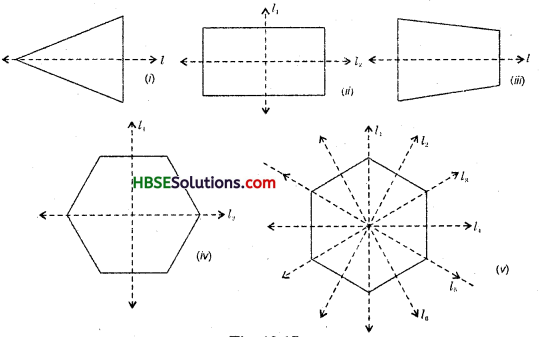Question 6.
Trace each figure and draw the lines of symmetry, if any :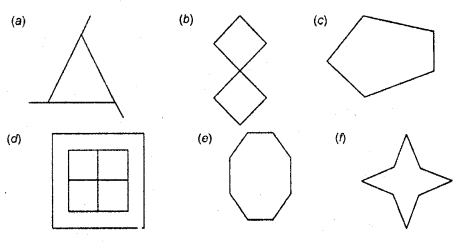Solution: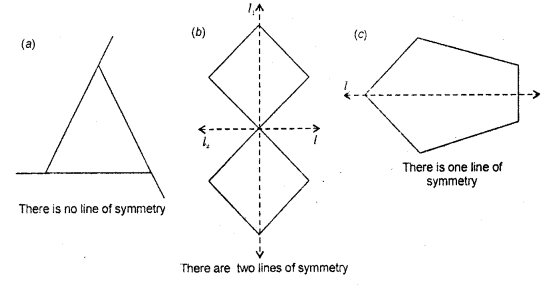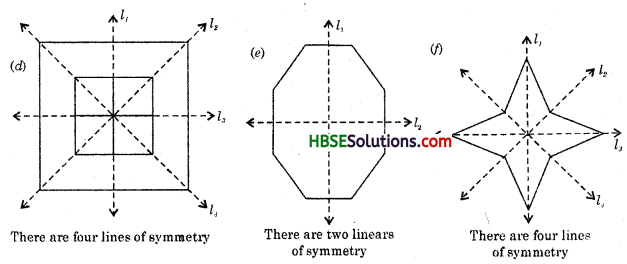Question 7.
Consider the English alphabet A to Z. List among them the letters which have
(a) vertical lines of symmetry, (like A)
(b) horizontal lines of symmetry, (like B)
(c) no line of symmetry (like Q)
Solution:
(a) Letters having vertical lines of symmetry are :
A, H, M, 0, T, U, V, W, X.
Cb) Letters having horizontal lines of symmetry are:
B, D, E, H, I, K, 0, X.
(c) Letters having no line of symmetry are :
C, F, G, J, L, N, P, Q, R, S, Y, Z.

Question 8.
Given here are figures of a few folded sheets and designs drawn about the fold. In each case, draw a rough diagram of the complete figure that would be seen when the design is cut off.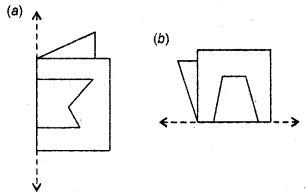Solution: